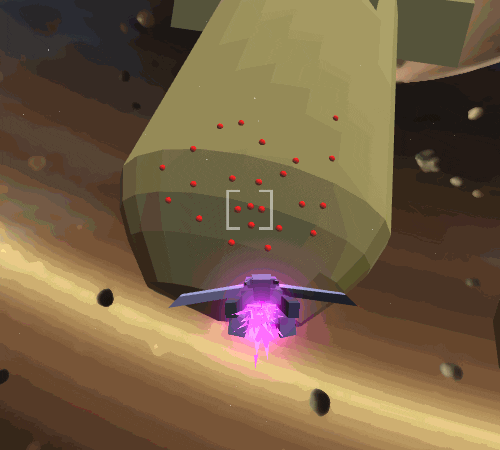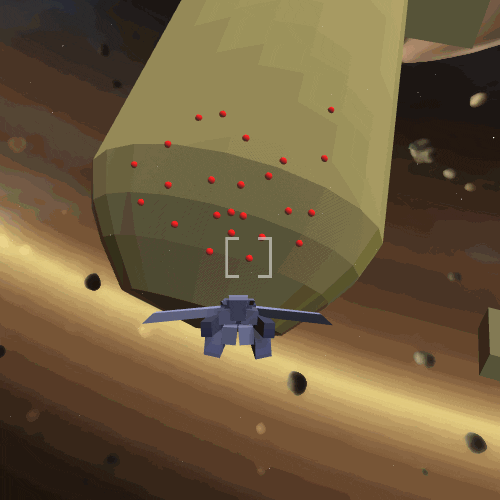# 🎮 Little Polygon Game Dev Blog

I designed and prototyped the missile attack! The math was clever and I want to show-off!

Let’s talk about cubic bezier curves, perlin noise, and rotation minimizing frames.Doing Ichirō Itano proud.

I’ll keep this article a little lighter on code. I really want to focus instead on the geometry. Many people are intimidated by math, but keep in mind that you don’t need to understand everything to use it.

Even if this is retreading topics you’ve already mastered, hopefully my own solution shows you some new ways you can combine these techniques creatively.

# Iterative vs. Closed-Form Motion

Broadly speaking, there are two flavors of movement code. Iterative Code updates an object position incrementally one frame at a time, in a process college-professors call integration. A common example of this Euler’s Method where we compute the velocity of the object and then nudge the position in that direction over a timestep:

``````void Update( float DeltaTime ) {
Vector3 Velocity = CalculateVelocity();
Vector3 Position = GetPosition();
SetPosition( Position + DeltaTime * Velocity );
}
``````

Delta is just ‘math speak’ for “change in” as in “the change in time this Update()”.

This is the natural way to write character control, in which the player input varies every frame, or complex physics simulations where there is no known realtime analytical solution.

Alternatively, if you know the whole motion ahead of time, you can use Closed-Form Code where you plot the whole path from initial conditions (math heads call it a parametric curve), and sample the current time. A good example of this is the well-known Cubic Bezier Curve:

``````Vector3 CalcBezierPos( Vector3 P0, Vector3 P1, Vector3 P2, Vector3 P3, float t ) {
float t_ = 1 - t;
return
(t_ * t_ * t_)    * P1 +
(3 * t_ * t_ * t) * P2 +
(3 * t_ * t * t)  * P3 +
(t * t * t)       * P4 ;
}
``````If you’ve ever used any vector graphics tool you probably recognize this. Beziers are cubic polynomials which is a fancy way of saying the simplest path with four degrees of freedom: the endpoints P0 and P3 and the “control points” P1 and P2 which affect the orientation and curvature.

The input t is called the input parameter and is a ratio on the range 0-1. So e.g. t=0.333 is about a third of the way through. To move a point, we simply take the elapsed time since the start of the motion, divided by the duration.

``````float StartTime;
float Duration;

void Update() {
float CurrentTime = Time.time;
float Elapsed = CurrentTime - StartTime;
if( Elapsed >= Duration )
SetPosition( P3 ); // we're at the end
else
SetPosition( CalcBezierPos( P0, P1, P2, P3, Elapsed / Duration ) );
}
``````

In addition to the position, we can also use the bezier parameters to calculate the derivative at t, which is the rate of change. This vector is useful because we say it’s tangent to the curve, i.e. it points in the direction of the motion. To convert this to speed, divide by the duration.

``````Vector3 CalcBezierDeriv( Vector3 P0, Vector3 P1, Vector3 P2, Vector3 P3, float t ) {
float t_ = 1 - t;
return  (
( 3 * t_ * t_ ) * ( P1 - P0 ) +
( 6 * t_ * t ) * ( P2 - P1 ) +
( 3 * t * t ) * ( P3 - P2 ) ;
}

float Velocity = CalcBezierDeriv( P0, P1, P2, P3, Elapsed / Duration ) / Duration;
``````Speed = Meters Per Second = ( Meters Per T ) / ( Seconds Per T ) = Deriv / Duration

# Simulating Homing Missiles

Because I know where the homing missile path starts (the launcher muzzle), and where it ends (the painted target), I chose to use a bezier curve as the base for the homing missile path.P1 is placed in front of the shooter, and P2 is projected out from the target surface.

Using a closed form kept things simple, because I didn’t have to solve a complicated “simulation” that hits the right point, and I can fine-tune the exact time between when you fire and when it hits, which is more intuitive than second-order physics units.This was a perfectly cromulent effect, if a bit bland. We can do better.

With a solid foundation, it’s time to start adding juice. Missile storm attacks in anime take erratic paths with more dynamism. We can simulate this by adding noise.

A common go-to for FX artists is Perlin Noise, a kind-of pseudorandom oscillation – it’s erratic, but also smooth. The code’s a little too long to post here, but it’s not hard to find samples online.Search for “Simplex Noise” (the name of a common optimized variant).

An obvious problem here is that I need the offset to be zero at endpoints, so the missile lines up with the muzzle and the target. I achieved this by multiplying it by an envelope which is zero at the ends and one in the middle.How do we turn a noise function into a 3D curve-deformed offset? We compute two independent noise values and use them as the X and Y components of an offset vector transformed by a rotation-frame that’s aligned to the bezier’s derivative (what a mouthful!).

``````Vector3 LocalOffset;
float NoiseFreq = 2f; // tuning value for wiggle frequency
float NoiseAmp = 8;   // tuning value for wiggle size
float Envelope = 1 - (1 - 2 * t) * (1 - 2 * t);
LocalOffset.x = NoiseAmp * Envelope * Noise( NoiseSeedX, NoiseFreq * Elapsed );
LocalOffset.y = NoiseAmp * Envelope * Noise( NoiseSeedY, NoiseFreq * Elapsed );
LocalOffset.z = 0;
Quaternion Frame = Quaternion.LookRotation( CalcBezierDeriv( P0, P1, P2, P3, t ) );
SetPosition( CalcBezierPos( P0, P1, P2, P3, t ) + Frame * LocalOffset );
``````TL;DR We’re wiggling along the red and green arrows.

This almost worked, but I saw some glitches. Vertically-Locked rotation frames calculated this way are aligned to the derivative, but twist a lot – especially when the path is vertical. Instead, we want so-called Rotation Minimizing Frames which have no instantaneous twist, just minimal swings between directions.(A) Vertically-Locked Frames (B) Rotation Minimizing Frames

The method of computing minimizing frames is mathematically complex in general, but luckily a paper was published in 2006 which described a shockingly simple method for “nudging” a frame forward without twisting called the Double Reflection Method. We don’t need to understand the derivation, we can just know that it’s cheap and it works.Shoutout to Jasper St. Pierre for showing me this mathy-ass math.

``````Quaternion Frame; // Initialize to Quaternion.LookDirection( P1 - P0 );

void UpdateFrame( float t ) {
// starting "normal" and "tangent"
var n0 = Frame * Vector3.up;
var t0 = Frame * Vector3.forward;

// target "tangent"
var t1 = CalcBezierDeriv( P0, P1, P2, P3, t ).normalized;

// first reflection
var v1 = CalcBezierPos( P0, P1, P2, P3, t ) - GetPosition();
var c1 = v1.sqrMagnitude;
var n0_l = n0 - (2 / c1) * Vector3.Dot(v1, n0) * v1;
var t0_l = t0 - (2 / c1) * Vector3.Dot(v1, t0) * v1;

// second reflection
var v2 = t1 - t0_l;
var c2 = v2.sqrMagnitude;
var n1 = n0_l - (2 / c2) * Vector3.Dot(v2, n0_l) * v2;

// build rotation with target normal for "up"
Frame = Quaternion.LookRotation( t1, n1 );
}
``````Et Voilà!

Thanks for reading! I promise I’ll do an art post next to give everyone a break from code 🙏